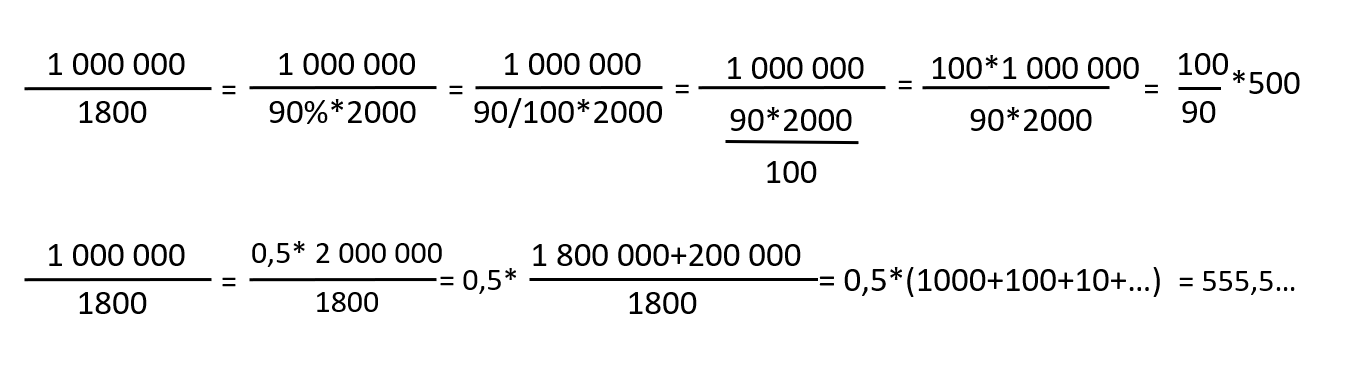# Math trick

case math Division
New answer on Nov 12, 2020
1.1 k Views

Hi,

I am looking for a trick that could help me to solve the following type of division fast:

1,000,000 / 1,800

What methods would you recommend?

Thank you!

A.

• Date ascending
• Date descending

1,000,000 / 1,800 = 1,000,000 / (2,000 / 1.1) = (1,000,000 / 2,000) * 1.1 = 500 * 1.1 = 550

The first one is a bit of a simplification that introduces a small error, but unless the interviewer asks you specifically to calculate this precicely, this should be fine.

Hi Agata,

Great question!

1. Cross out 0s
1. 10,000/18
2. Simplify
1. 5,000/9 (cut in half)
3. If rounding, get to closest, and add a segment
1. 5,000/10 = 500
2. It's a bit less (1/10), so add another 50
3. = approx 550
4. If you're not allowed to round, long division

What's that you say? I may have to learn long division and long multiplication? Yes. 100% :)

This is so easy, thank you!

Personnally, I always go to things i know well. For instance here, 1 millions is half of 2 millions so you could go with two millions divided by 1800 and take half of your result.

Another way is to realize that 1800 is only 10% smaller than 2000. 1800= 90% *2000=90/100*2000.

So 1 000 000/1800 is actually 100/90* 1 000 000/2000=100/90*500.

Then it's easy because 100/90=10/9=1.11.
So 1 000 000/1800= 1.11*500=555.

Here i detailed all step but this can be done much easier once you realized that you can use 2000 and go from that.

Here is the detailled version for both calculation(edited)

Thank you! This sounds straightforward, but I don't understand this bit: So 1 000 000/1800 is actually 100/90* 1 000 000/2000=100/90*500. Why do we have 100/90? 90% is 90/100. But if I put 90/100 the result is wrong...

Hi Agatha, I added all the steps for both method to make my answer more understandable. Hope it helps.

Thank you!

Hello!

I would divide into 2000 instead. Then remove zeros, and simple division.

Once it´s done, make it ~20% higher for the simplificaiton we made.

Best,

Clara

Hi Agata,

There are plenty of threads and tons of tips and prep guidance for maths. Please search the forum. Have a look at these:

• https://www.preplounge.com/en/consulting-forum/moltiplicationsdivisions-with-6-digits-numbers-estimation-math_tricks-2925
• https://www.preplounge.com/en/consulting-forum/best-apps-for-mental-maths-4376
• https://www.preplounge.com/en/consulting-forum/case-math-practice-6877